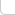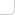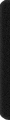# 某岛

… : "…アッカリ～ン . .. . " .. .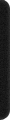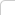February 16, 2013

## Problem C. Secret

### Brief description:

1~n 分成 k 份，使得 k 份都不是等差数列。。
（。。1-k 1-k k-1 1 1 1 1 1 1 1 。。。）

## Problem D. Good Substrings

### Brief description:

。。给定一个长度为 n 字符串。。每个字符有 好/坏 两种属性。。（ n <= 1,500 一个字串被称为好的。。如果其中坏字符 <= k 个。。问不同的好子串有多少个。。 太可怕了…… 卡掉字典序hash的方法 by xhr

## Problem E. Three Horses

### Brief description:

。三种操作。。

• (a, b) -> (a+1, b+1) | 平移。。
• (a, b) -> (a/2, b/2) | 折半。。a, b is even
• (a, b), (b, c) -> (a, c) | 连接。。

。。问有多少初始操作 (a, b)，满足 1 <= a < b <= m，使得可以通过上述操作生成。。 。。(1, a1), (1, a2), ..., (1, an) 这些二元组。。

### Analysis:

。。因此若只有 a1 时。。设 x = a1 -1 。。

。。枚举每个约数。。累计 f(x) 即可。。

LL f(int x){
if (!(x&1)) return 0;
LL res = 0; for (int i=0;x<<i<m;++i) res += m-(x<<i);
return res;
}


。。多个 ai 。。。则开始需要对他们取 gcd 。。。

//}/* .................................................................................................................................. */

int n, m;

LL f(int x){
if (!(x&1)) return 0;
LL res = 0; for (int i=0;x<<i<m;++i) res += m-(x<<i);
return res;
}

int main(){

#ifndef ONLINE_JUDGE
freopen("in.txt", "r", stdin);
//freopen("out.txt", "w", stdout);
#endif

RD(n, m); int d = 0; REP(i, n) d = __gcd(d, int(RD()-1));
int t = sqrt(d); LL res = t*t == d ? -f(t) : 0;
REP_1(i, t) if (d%i==0) res += f(i) + f(d/i);

OT(res);
}